# pattern

Plot 3-D radiation pattern of antenna

## Syntax

``pat = pattern(tx)``
``pat = pattern(rx,freq)``
``pat = pattern(___,Name,Value)``

## Description

example

````pat = pattern(tx)` plots the 3-D radiation pattern of the antenna for each transmitter in vector `tx`. The signal gain value (in dBi) in a particular direction determines the color of the pattern. The function scales the pattern on the plot according to the `Size` name-value argument. The function plots the pattern for the transmitter frequency as specified by the `Frequency` property of `tx`.```

example

````pat = pattern(rx,freq)` plots the 3-D radiation pattern of the antenna for each receiver in vector, `rx` with frequency `freq`.```
````pat = pattern(___,Name,Value)` specifies options using one or more name-value arguments in addition to any of the input argument combinations in previous syntaxes. For example, `'ColorMap','jet'` specifies the jet colormap for coloring the pattern plot.```

## Examples

collapse all

Set up the satellite scenario.

```startTime = datetime(2021,2,12,13,30,0); stopTime = startTime + hours(5); sampleTime = 60; %seconds sc = satelliteScenario(startTime,stopTime,sampleTime);```

Create a satellite, ground station, transmitter, and receiver.

```sat = satellite(sc,1e7,0,0,0,0,0); gs = groundStation(sc,"Latitude",30,"Longitude",74); tx = transmitter(sat,"Frequency",3e9); rx = receiver(gs);```

Visualize the scenario in the satellite scenario viewer.

`viewer = satelliteScenarioViewer(sc);`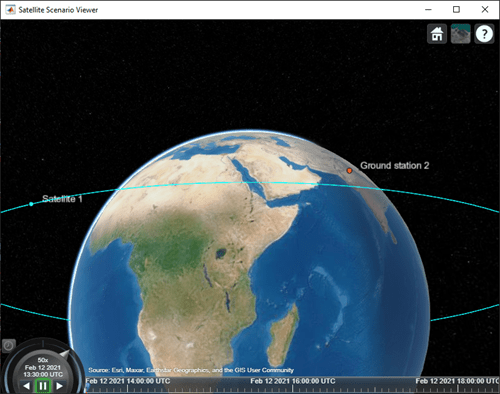Plot the radiation pattern of the transmitter antenna.

`pat = pattern(tx);`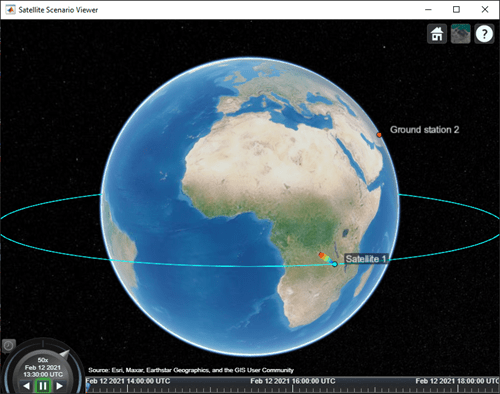Point the satellite at the ground station. The pattern rotates to reflect the new orientation of the antenna.

`pointAt(sat,gs);`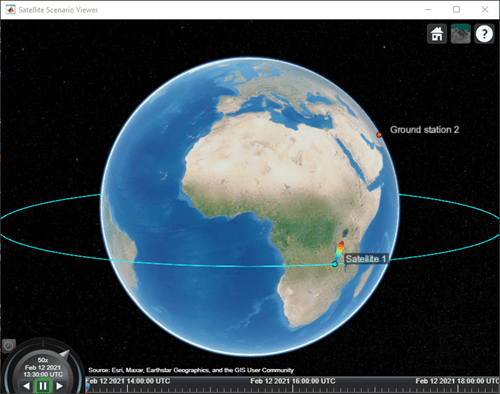Increase the visual size of the radiation pattern.

```pat.Size = 3000000; pat.Colormap = "parula";```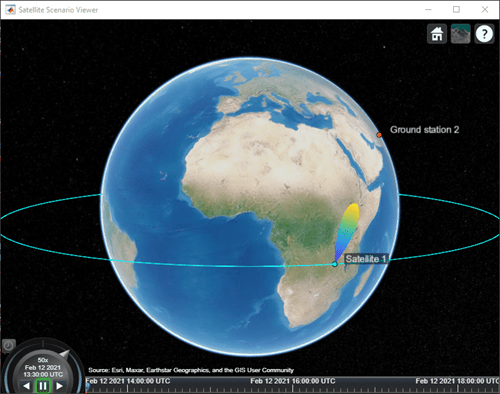Set up the satellite scenario.

`sc = satelliteScenario;`

Create a satellite, ground station, transmitter, and receiver.

```sat = satellite(sc,1e7,0,0,0,0,0); gs = groundStation(sc,"Latitude",30,"Longitude",74); tx = transmitter(sat,"Frequency",1e9); rx = receiver(gs);```

Visualize the scenario in the satellite scenario viewer.

`viewer = satelliteScenarioViewer(sc);`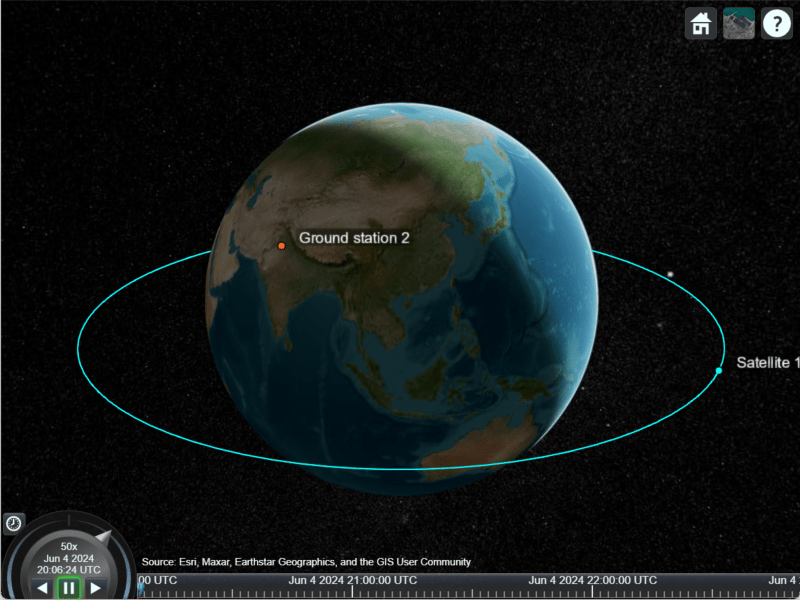```freq = 30e9; pat = pattern(rx,freq);```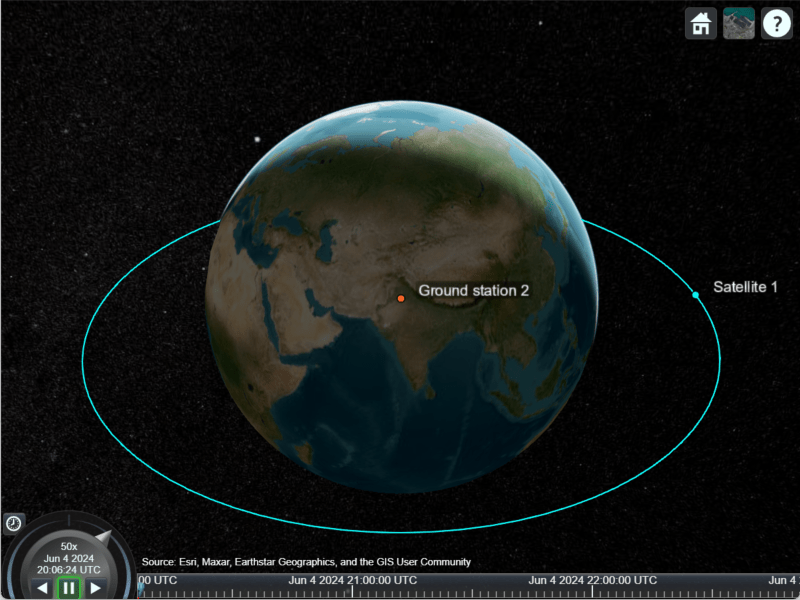Increase the visual size and specify the transparency of the radiation pattern.

```pat.Size = 3000000; pat.Colormap = 'autumn';```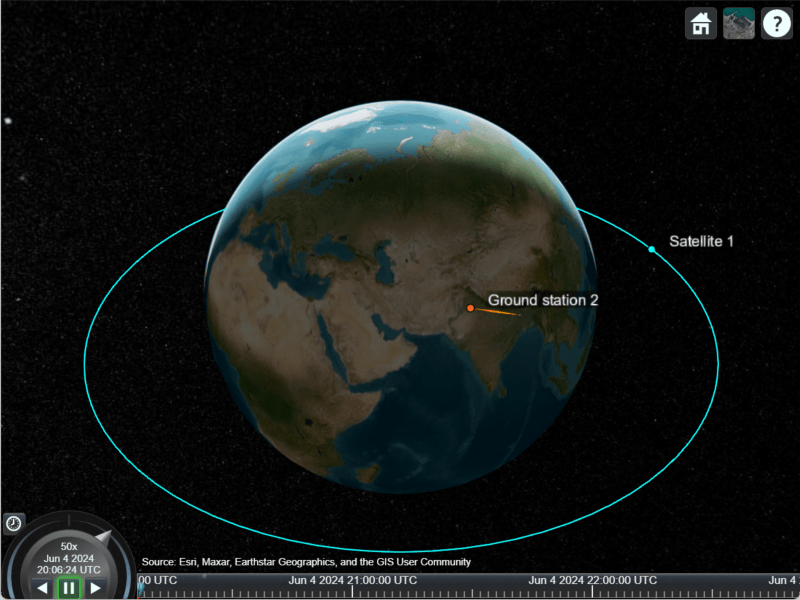## Input Arguments

collapse all

Transmitter object, specified as either a scalar or vector.

Receiver object, specified as either a scalar or vector.

Frequency to calculate radiation pattern, specified as a scalar or a vector.

• If `freq` is scalar, its value is applied to the pattern of all receivers in `rx`.

• If `freq` is vector, its length must equal to that of `rx`.

Each element in `freq` corresponds to the pattern of the antenna of the receiver in `rx`.

Data Types: `double`

### Name-Value Arguments

Specify optional pairs of arguments as `Name1=Value1,...,NameN=ValueN`, where `Name` is the argument name and `Value` is the corresponding value. Name-value arguments must appear after other arguments, but the order of the pairs does not matter.

Before R2021a, use commas to separate each name and value, and enclose `Name` in quotes.

Example: `'Size'`,`1000` sets the size of the radiation pattern plot to 1,000 meters.

Size of the radiation pattern plot, specified as a numeric scalar in meters. This value represents the distance between the antenna position and the point on the plot with the highest gain.

Data Types: `double`

Colormap for coloring the pattern plot, specified as a predefined colormap name or an M-by-3 matrix of red, green, blue (RGB) triplets that define M individual colors. For more information on the colormap names, see map.

Data Types: `double` | `char` | `string`

Transparency of the pattern plot, specified as a scalar in the range [0, 1]. A value of `0` means the plot is completely transparent, and a value of `1` means the plot is opaque.

Data Types: `double`

Resolution of the 3-D pattern, specified as `'low'`, `'medium'`, or `'high'`. Use this argument to control the visual quality of the pattern and time the function takes to plot the pattern. `'low'` corresponds to the fastest and least-detailed pattern.

Data Types: `char` | `string`

Satellite Scenario Viewer to visualize the satellite, specified as a scalar, row vector, or matrix of `satelliteScenarioViewer` objects that are associated with the satellite scenario.

## Output Arguments

collapse all

Radiation pattern visualization for transmitter or receiver object, returned as either a scalar or vector.

## Version History

Introduced in R2021b